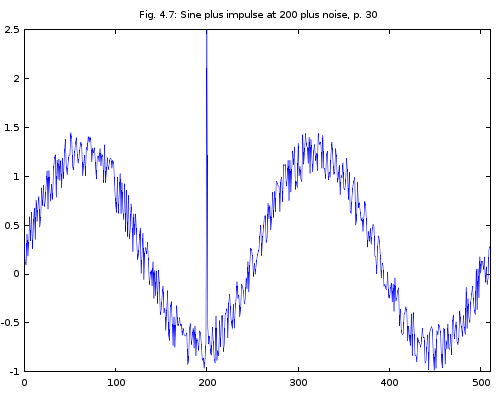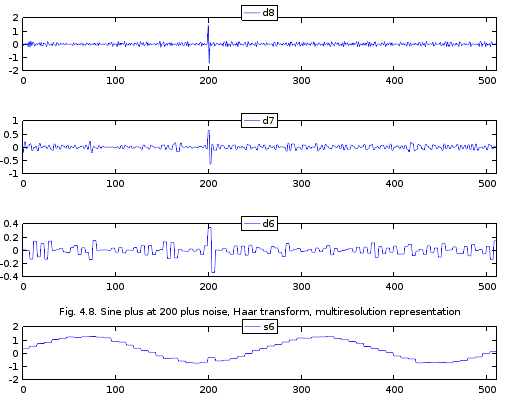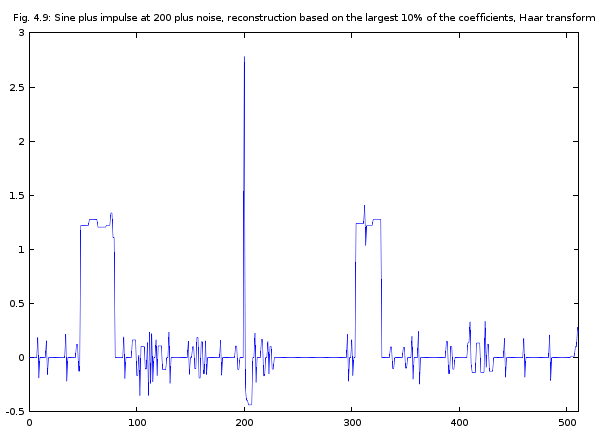## Monday, September 28, 2015

### Noise Reduction in the 3-Scale Ordered HWT Representation of a Synthetic Sinusoid with Added Random Noise: Programmatic Note 5 in Ripples in Mathematics

Introduction

This is my programmatic note 5 on Ch. 04, p. 29, in "Ripples in Mathematics" by A. Jensen & A. la Cour-Harbo. May the beauty and harmony of this text be conveyed to the readers of this post. These notes document my programmatic journey, sometimes easy, sometimes painful but always joyful, through this text. These notes are written for those who want to use Java as a programming investigation tool of various wavelet algorithms and Octave to plot the results. You can find my previous notes by searching my blog on "ripples in mathematics." If you are on the same journey, let me know of any bugs in my code or improvements you have found that let us get the results in a better way.

The problem addressed in this post is formulated at  the bottom of p. 28 in "Ripples in Mathematics." The problem is to reduce noise in a synthetic signal by processing its 3-scale ordered HWT representation. The signal is generated by the sinusoid y = sin(4*pi*t), for t in [0, 1], sampled at 512 equidistant points. The the value of the 200th sample is set to 2, and some random noise is added into the sample.

The removal of noise is accomplished by first applying the 3-scale ordered HWT to the signal to obtain the transform s6, d6, d7, d8. You can look at this post for a sketchy explanation of the notation s6, d6, d7, d8. In this transformed representation, the largest 10% of the coefficients are left unchanged while the other coefficients are set to 0. Then the 3-scale inverse ordered HWT is applied to this modified transform in the hope that the introduced random noise is removed from the signal.

The sections below describe what I have done to compute each step and then plot it.

Introduction of Small Random Noise in Java

To the best of my understanding, the authors do not give the exact formula they used to add a small random noise to the sinusoid. So, I wrote a static method in RipplesInMathCh04.java that adds noise to a signal represented as an array of doubles. A possible improvement on this method is to abstract the value by which each Math.random() is divided as an argument.

public static void addNoiseToSignal(double[] signal) {
for(int i = 0; i < signal.length; i++) {
signal[i] += Math.random()/2.0;
}

}

I then implemented a method that outputs the numbers for Figure 4.7 on p. 30 in my copy of "Ripples in Mathematics." The source of Ripples_F_p25 is here.

static Ripples_F_p25 sRipples_F_p25 = new Ripples_F_p25();
static void fig_4_7_p30() {
for(int i = 0; i < 512; i++)  {
sRange[i] = sRipples_F_p25.v(sDomain[i]);
}

sRange = 2; // spike at 200
display_signal(sRange);

}

I ran the above method and placed its output to a Octave script fig_4_7_p30.m to plot the sinusoid with the random noise and the spike at 200. The output of my Octave script is given in Figure 1.Figure 1. Plot generated by fig_4_7_p30.m

Applying 3-Scale Ordered HWT to the Signal with Random Noise

To apply the 3-scale ordered HWT to the signal with the random noise and a spike of 2 at 200 I implemented the five methods below in RipplesInMathCh04.java.  The method multires_fig_4_8_p30() is a workhorse used by the four methods defined after it to compute the data for each of the four the graphs in Figure 4.8 on p. 30 in my copy of "Ripples in Mathematics."

static void multires_fig_4_8_p30(String message, int range_start, int range_end) {
for(int i = 0; i < 512; i++)  { sRange[i] = sRipples_F_p25.v(sDomain[i]); }

sRange = 2; // spike at 200

OneDHaar.orderedNormalizedFastHaarWaveletTransformForNumIters(sRange, 3);

double[] signal = new double[sRange.length];
for(int i = 0; i < 512; i++) {
if ( i >= range_start && i <= range_end ) {
signal[i] = sRange[i];
}
else {
signal[i] = 0;
}
}

System.out.println("=========================");
System.out.println(message);
display_signal(signal);
System.out.println("=========================");
OneDHaar.orderedNormalizedFastInverseHaarWaveletTransformForNumIters(signal, 3);
display_signal(signal);
System.out.println("=========================");

}

// d8 range values for Fig. 4.8, p. 30 in "Ripples in Mathematics."
static void fig_4_8_s06_d06_d07_d8_p30() { multires_fig_4_8_p30("06-06-07-d8, Fig. 4.8, p. 30", D8_START, D8_END); }
// d7 range values for Fig. 4.8, p. 30 in "Ripples in Mathematics."
static void fig_4_8_s06_d06_d7_d08_p30() { multires_fig_4_8_p30("06-06-d7-08, Fig. 4.8, p. 30", D7_START, D7_END); }
// d6 range values for Fig. 4.8, p. 30 in "Ripples in Mathematics."
static void fig_4_8_s06_d6_d07_d08_p30() { multires_fig_4_8_p30("06-d6-07-08, Fig. 4.8, p. 30", D6_START, D6_END); }
// s6 range values for Fig. 4.8, p. 30 in "Ripples in Mathematics."
static void fig_4_8_s6_d06_d07_d08_p30() { multires_fig_4_8_p30("s6-06-07-08, Fig. 4.8, p. 30", S6_START, S6_END); }

I placed the outputs of these methods into an Octave script fig_4_8_p30.m. The plot computed by the script is given in Figure 2.Figure 2. Plot generated by fig_4_8_p30.m

Inversing the 3-Scale ordered HWT with the Largest 10% Coefficients Kept Intact

The removal of the random noise starts by keeping the largest 10% of the coefficients in the 3-scale ordered HWT of the signal with random noise. I wrote the method process_signal_range() in that takes a sub-range in a signal and keeps the percent of the largest coefficients in that range. The other coefficients are set to 0.

static void process_signal_range(double[] signal, int range_start, int range_end, double percent) {
final int range_length = range_end - range_start + 1;
double[] sorted_range = new double[range_length];
// copy the signal segment into sorted_range
System.arraycopy(signal, range_start, sorted_range, 0, range_length);
Arrays.sort(sorted_range); // sort the range
final int len = (int) (range_length * (percent/100.0));
final int sorted_range_min_index = Math.max(0, range_length - len - 1);

if ( sorted_range_min_index > range_length - 1 ) {
System.out.println("range cannot be discretized");
return;
}
final double sorted_range_min = sorted_range[sorted_range_min_index];
// set all values in signal less than the sorted_range_min to 0.0
for(int i = range_start; i < range_end; i++) {
if ( signal[i] < sorted_range_min ) {
signal[i] = 0.0;
}
}

}

The method fig_4_9_p31() in produces the data for Figure 4.9 on p. 31 in my copy of "Ripples in Mathematics."

static void fig_4_9_p31() {
for(int i = 0; i < 512; i++)  {
sRange[i] = sRipples_F_p25.v(sDomain[i]);
}

sRange = 2; // spike at 200
System.out.println("=========================");
System.out.println("Signal with Noise:");
display_signal(sRange);

OneDHaar.orderedNormalizedFastHaarWaveletTransformForNumIters(sRange, 3);

double percent = 10.0;
process_signal_range(sRange, D8_START, D8_END, percent);
process_signal_range(sRange, D7_START, D7_END, percent);
process_signal_range(sRange, D6_START, D6_END, percent);
process_signal_range(sRange, S6_START, S6_END, percent);

System.out.println("=========================");
System.out.println("Processed Signal with Noise:");
display_signal(sRange);
System.out.println("=========================");
System.out.println("Inversed Signal with Noise:");
OneDHaar.orderedNormalizedFastInverseHaarWaveletTransformForNumIters(sRange, 3);
display_signal(sRange);
System.out.println("=========================");
}

I placed the output of fig_4_9_p31() in my Octave script fig_4_9_p31.m. The plot is given in Figure 3. It is in the same shape and form as the curve in the book. It is possible to recognize the spike at 200 and the general form of the sine signal but the reconstructed signal is does not quite resemble the noisy signal.Figure 3. Output of fig_4_9_p31.m